#### Using the plan

Assume that there is no limit on the number of stages. After the value iterations terminate, cost-to-go functions are determined over. This is not exactly a plan, because an action is required for each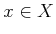. The actions can be obtained by recording the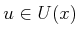that produced the minimum cost value in (10.45) or (10.39).

Assume that the value iterations have converged to a stationary cost-to-go function. Before uncertainty was introduced, the optimal actions were determined by (2.19). The nondeterministic and probabilistic versions of (2.19) are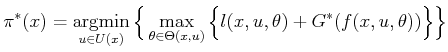(10.48)

and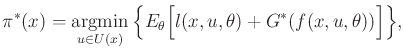(10.49)

respectively. For eachat which the optimal cost-to-go value is known, one evaluation of (10.45) yields the best action.

Conveniently, the optimal action can be recovered directly during execution of the plan, rather than storing actions. Each time a state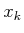is obtained during execution, the appropriate action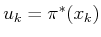is selected by evaluating (10.48) or (10.49) at. This means that the cost-to-go function itself can be interpreted as a representation of the optimal plan, once it is understood that a local operator is required to recover the action. It may seem strange that such a local computation yields the global optimum; however, this works because the cost-to-go function already encodes the global costs. This behavior was also observed for continuous state spaces in Section 8.4.1, in which a navigation function served to define a feedback motion plan. In that context, a gradient operator was needed to recover the direction of motion. In the current setting, (10.48) and (10.49) serve the same purpose.

Steven M LaValle 2020-08-14